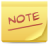Hierarchy

Clustering can be stored inside a hierarchy of nodes.

There are 2 ways to write a hierarchy in GEXF, depending on how you process your data:

• Sequential-safe Reading: Nodes can simply host other nodes and so on.
• Random Writing: Each node refer to a parent node id with the XML-attribute pid.

The frst style is preferred when the structure written is previously ordered. Sequential reading of this kind of GEXF is safe because no node reference is used. But in the case your program can't provide it, the second way allows writing (and then reading) nodes randomly, but linear reading is at your own risk.

This graph represents a hierarchy of people:

http://gexf.net/data/hierarchy1.gexf
<?xml version="1.0" encoding="UTF-8"?>
<gexf xmlns="http://www.gexf.net/1.2" xmlns:xsi="http://www.w3.org/2001/XMLSchema-instance" xsi:schemaLocation="http://www.gexf.net/1.2 http://www.gexf.net/1.2/gexf.xsd" version="1.2">
<graph mode="static" defaultedgetype="directed">
<nodes>
<node id="a" label="Kevin Bacon">
<nodes>
<node id="b" label="God">
<nodes>
<node id="c" label="human1"/>
<node id="d" label="human2"/>
</nodes>
</node>
<node id="e" label="Me">
<nodes>
<node id="f" label="frog1"/>
<node id="g" label="frog2"/>
</nodes>
</node>
</nodes>
</node>
</nodes>
<edges>
<edge id="0" source="b" target="e" />
<edge id="1" source="c" target="d" />
<edge id="2" source="g" target="b" />
<edge id="3" source="f" target="a" />
</edges>
</graph>
</gexf>

If you can't structure your graph topology before writing a GEXF file, you may use the second style. Nodes sent to Gephi from a live data source, e.g. a web crawler, are written like this. Note that edges are always written randomly.

This is the same graph:

http://gexf.net/data/hierarchy4.gexf
<?xml version="1.0" encoding="UTF-8"?>
<gexf xmlns="http://www.gexf.net/1.2" xmlns:xsi="http://www.w3.org/2001/XMLSchema-instance" xsi:schemaLocation="http://www.gexf.net/1.2 http://www.gexf.net/1.2/gexf.xsd" version="1.2">
<graph mode="static" defaultedgetype="directed">
<nodes>
<node id="g" label="frog2" pid="e" />
<node id="a" label="Kevin Bacon" />
<node id="c" label="human1" pid="b" />
<node id="b" label="God" pid="a" />
<node id="e" label="Me" pid="a" />
<node id="d" label="human2" pid="b" />
<node id="f" label="frog1" pid="e" />
</nodes>
<edges>
<edge id="0" source="b" target="e" />
<edge id="1" source="c" target="d" />
<edge id="2" source="g" target="b" />
<edge id="3" source="f" target="a" />
</edges>
</graph>
</gexf>

Get more details on how to write different styles of hierarchy in the Primer.

See how to transform a hierarchical to a phylogenetic graph in the next example.Hierarchies are not visualized as trees in Gephi, but are interpreted as meta-clusters.